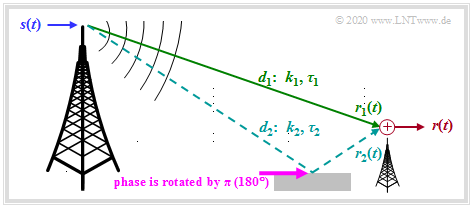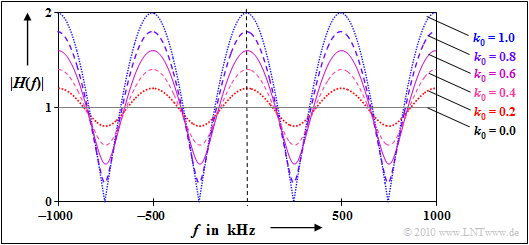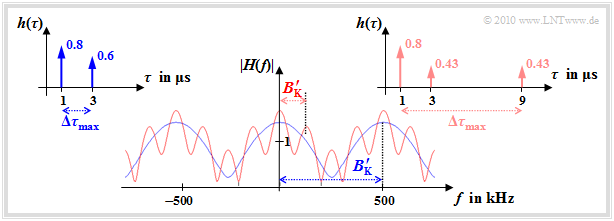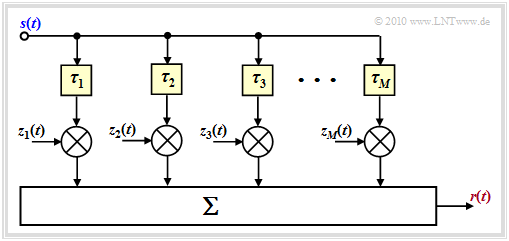# Multi-Path Reception in Mobile Communications

## Time-invariant description of the two-way channel

We assume the scenario shown in the graph.  This assumesTime–invariant consideration of the two-way channel
• Transmitter and receiver are  at rest
Then both the channel transfer function and the impulse response are time–independent.  For all times  $t$  applies  $H(f, \hspace{0.05cm}t) = H(f)$  and  $h(\tau, \hspace{0.05cm}t) = h(\tau)$.
• two-way channel:
The transmission signal  $s(t)$  reaches the receiver on a direct path with the path length  $d_1$,  and there is also an echo due to the reflective ground  $($the total path length is  $d_2)$.

Thus, the following applies to the received signal:

$r(t) = r_1(t) + r_2(t) = k_1 \cdot s( t - \tau_1) + k_2 \cdot s( t - \tau_2) \hspace{0.05cm}.$

The following statements should be noted:

• Compared to the transmission signal, the signal  $r_1(t)$  received via the direct path is attenuated by the factor  $k_1$  and delayed by   $\tau_1$ .
• The attenuation factor  $k_1$  is calculated with the  path loss model.  The greater the transmission frequency  $f_{\rm S}$,  the distance  $d_1$  and the exponent  $\gamma$  are, the smaller is   $k_1$  and thus the greater is the loss.
• The delay  $\tau_1 = d_1/c$  increases proportionally with the path length  $d_1$ .   For example, for the distance  $d_1 = 3 \ \rm km$  and the speed of light  $c = 3 \cdot 10^8 \ \rm m/s$  the delay will be  $\tau_1 = 10 \ \rm µ s$.
• Because of the larger path length  $(d_2 > d_1)$  the second path has a greater attenuation   ⇒   smaller pre-factor   ⇒   $(|k_2| < |k_1|)$  and accordingly also a greater delay   $(\tau_2 > \tau_1)$.
• In addition, it must be taken into account that the reflection from buildings or the ground leads to a phase rotation of  $\pi \ (180^\circ)$.  This causes the factor  $k_2$  to become negative.  In the following, however, the negative sign of  $k_2$  is ignored.

Note:   We refer here to the SWF applet  Multipath propagation and frequency selectivity  (German language!).

## Simple time–invariant model of the two-way channel

For the frequency selectivity

• the path loss  $($marked by  $k_1)$  and
• the basic term  $\tau_1$

are irrelevant. The only decisive factors here are path loss differences and runtime differences.

We will now describe the two-way channel with the new parameters

$$k_0 = |k_2 /k_1 |,\hspace{0.5cm} \tau_0 = \tau_2 - \tau_1.$$

This results in:

$r(t) = r_1(t) + k_0 \cdot r_1( t - \tau_0) \hspace{0.5cm}{\rm with} \hspace{0.5cm} r_1(t) = k_1 \cdot s( t - \tau_1)\hspace{0.05cm}.$

The figure illustrates the equation.  With the simplifications  $k_1 = 1$  and  $\tau_1 = 0$    ⇒   $r_1(t) = s(t)$  we obtain:

$r(t) = s(t) + k_0 \cdot s( t - \tau_0) \hspace{0.05cm}.$

From this simplified model  (without the grey-shaded block)  important descriptive variables can be easily calculated:

$H(f) = {R(f)}/{S(f)} = 1 + k_0 \cdot {\rm e}^{ - {\rm j} \hspace{0.05cm}\cdot \hspace{0.05cm}2 \pi f \hspace{0.05cm} \cdot \hspace{0.05cm} \tau_0} \hspace{0.05cm}.$
$h(\tau) = 1 + k_0 \cdot \delta(\tau - \tau_0) \hspace{0.05cm}.$

$\text{Example 1:}$  We consider a two-way channel with delay   $\tau_0 = 2 \ \ \rm µ s$  and some attenuation factors  $k_0$  between  $0$  and  $1$.Absolute value of the transfer function of a two-way channel   $(\tau_0 = 2 \ \rm µ s)$

The graph shows the transfer function in terms of its absolute value in the range  $\pm 1 \ \rm MHz$.  You can see from this representation:

• The transfer function  $H(f)$  and also its absolute value is periodic with  $1/\tau_0 = 500 \ \rm kHz$.
• The fluctuations around the mean value  $\vert H(f) \vert = 1$  are the stronger, the larger the  (relative)  contribution  $k_0$  of the second path is  (i.e. the echo).

## Coherence bandwidth as a function of M

We are now modifying the two-way model in such a way that we allow more than two paths, as is the case for mobile communications.Frequency response at  $M = 2$  (blue) and  $M = 3$  (red)

In general, the multipath channel model is thus:

$$r(t)= \sum_{m = 1}^{M}\hspace{0.15cm} k_m \cdot s( t - \tau_m)$$
$$\Rightarrow \hspace{0.3cm} h(\tau) = \sum_{m = 1}^{M}\hspace{0.15cm} k_m \cdot \delta( \tau - \tau_m) \hspace{0.05cm}.$$

We now compare

• the  "two-way channel"  $(M = 2)$  with the parameters
$\tau_1 = 1\,\,{\rm µ s}\hspace{0.05cm}, \hspace{0.2cm} k_1 = 0.8\hspace{0.05cm}, \hspace{0.2cm} \tau_2 = 3\,\,{\rm µ s}\hspace{0.05cm}, \hspace{0.2cm} k_2 = 0.6$
• and the following  "three-way channel"  $(M = 3)$:
$$\tau_1 = 1\,\,{\rm µ s}\hspace{0.05cm}, \hspace{0.2cm} k_1 = 0.8\hspace{0.05cm}, \hspace{0.2cm} \tau_2 = 3\,\,{\rm µ s}\hspace{0.05cm}, \hspace{0.2cm} k_2 \approx 0.43\hspace{0.05cm}, \hspace{0.2cm} \tau_3 = 9\,\,{\rm µ s}\hspace{0.05cm}, \hspace{0.2cm} k_3 \approx 0.43 \hspace{0.05cm}.$$

With the selected constants, both channels have the root mean square value  ${\rm E}\big [k_m^2\big ] = 1$.

The graph shows the magnitude functions  $|H(f)|$  of both channels and the corresponding impulse responses  $h(\tau)$.  One can see from these graphs:

• In the blue channel  $(M = 2)$  the Dirac functions occur in a range of width  $\Delta \tau_{\rm max} = 2 \ \rm µ s$.
• With the red channel  $(M = 3)$  this value is four times as large:   $\Delta \tau_{\rm max} = 8 \ \rm µ s$.
• As a first approximation for  coherence bandwidth  $B_{\rm K}\hspace{0.01cm}'\approx 1/ \Delta \tau_{\rm max}$ is often used, which may differ from the correct value by a factor of  $2$  or more.
• This simple approximation, marked with an apostrophe, results for the blue channel to  $B_{\rm K}\hspace{0.01cm}'= 500 \ \rm kHz$.
• For the red channel it is  $B_{\rm K}\hspace{0.01cm}'= 125 \ \rm kHz$  which is just one fourth of the blue channel's.

$\text{In general the following applies:}$

1.   If the signal bandwidth  $B_{\rm S} = 1/T_{\rm S}$  is much smaller than the coherence bandwidth  $B_{\rm K}$, then the channel can be considered as non-frequency selective
$(T_{\rm S}$  denotes the symbol duration$)$.
2.   In other words:   For a given  $B_{\rm S}$  the smaller the coherence bandwidth  $B_{\rm K}$  or the larger the maximum delay  $\Delta \tau_{\rm max}$  the greater the frequency selectivity.
3.   This also means:   The frequency selectivity is often determined by the longest echo.
4.   Many short echoes with a total energy  $E$  are less disturbing than one long echo of the same energy  $E$.

## Consideration of the time variance

Up to now the attenuation factors  $k_m$  were assumed to be constant.  For mobile radio, however, this channel model is only correct if transmitter and receiver are static, which is merely a special case for this communication system.

For a moving user, these constant factors  $k_m$  must be replaced by the time-variant factors  $z_m(t)$  which are each based on random processes. You should note this:Mobile channel model considering time variance and echoes
• The bindings within the process  $z_m(t)$  are related to the mobility properties  (speed, direction, etc.)  to the  Jakes Spectrum .

The figure shows the generally valid model for the mobile communications channel.  "Generally valid" but only with reservations, as explained at the end of  $\text{Example 2}$.

For an understanding of the figure we refer to the chapter  General description of the mobile communications channel. Please note:

• The  $M$  main paths are characterized by large propagation time differences.
• The time-variant complex coefficients  $z_m(t)$  result from the sum of many secondary paths whose delay times are all approximately the same  $\tau_m$ .

$\text{Example 2:}$  Studies have shown that in mobile communications no more than four or five main pathways are effective at the same time.

The represented 2D–impulse response  $h(\tau,\hspace{0.1cm} t)$  applies to  $M = 3$  main paths with time-variant behavior, where the received power decreases with increasing delay in the statistical average.  For this graph the above sketched channel model is used as a basis.

Two different views are shown:

• The left image shows  $h(\tau,\hspace{0.1cm} t)$  as a function of the delay time  $\tau$  at a fixed time  $t$.
• The viewing direction in the right image is rotated by  $90^\circ$ .  By using the color coding, the representation should be understandable.

This geaphic also shows the weak point of our mobile communications channel model:   Although the coefficients  $z_m(t)$  are variable, the delay times  $\tau_m$  are fixed.   This does not correspond to reality, if the mobile station is moving and the connection takes place in a changing environment.    $\tau_m(t)$  should be considered.

$\text{Conclusion:}$  It is helpful to make a slight modification to the above model:

• One chooses the number  $M'$  of (possible) main paths much larger than necessary and sets  $\tau_m = m \cdot \Delta \tau$.
• The incremental  (minimum resolvable)  delay  $\Delta \tau = T_{\rm S}$  results from the sampling rate and thus from the bandwidth  $B_{\rm S} = 1/T_{\rm S}$  of the signal  $s(t)$.
• The maximum delay time  $\tau_\text{max} = M' \cdot \Delta \tau$  of this model is equal to the inverse of the coherence bandwidth  $B_{\rm K}$.  The number of paths considered is thus  $M' = B_{\rm S}/B_{\rm K}$.

Here, too, usually no more than  $M = 5$  main paths simultaneously provide a relevant contribution to the impulse response.

• The advantage over the first model is that for the delays now all values  $\tau_m \le \tau_\text{max}$  are possible, with a temporal resolution of  $\Delta \tau$ .
• At the end of next chapter  The GWSSUS channel model  we will come back to this general model again.